The FORECAST Procedure

OUTEST= Data Set

The FORECAST procedure writes the parameter estimates and goodness-of-fit statistics to an output data set when the OUTEST= option is specified. The OUTEST= data set contains the following variables:

• the BY variables

• the first ID variable, which contains the value of the ID variable for the last observation in the input data set used to fit the model

• _TYPE_, a character variable that identifies the type of each observation

• the VAR statement variables, which contain statistics and parameter estimates for the input series. The values contained in the VAR statement variables depend on the _TYPE_ variable value for the observation.

The observations contained in the OUTEST= data set are identified by the _TYPE_ variable. The OUTEST= data set might contain observations with the following _TYPE_ values:

AR1–ARn

The observation contains estimates of the autoregressive parameters for the series. Two-digit lag numbers are used if the value of the NLAGS= option is 10 or more; in that case these _TYPE_ values are AR01–ARn. These observations are output for the STEPAR method only.

CONSTANT

The observation contains the estimate of the constant or intercept parameter for the time trend model for the series. For the exponential smoothing and the Winters’ methods, the trend model is centered (that is, t =0) at the last observation used for the fit.

LINEAR

The observation contains the estimate of the linear or slope parameter for the time trend model for the series. This observation is output only if you specify TREND=2 or TREND=3.

N

The observation contains the number of nonmissing observations used to fit the model for the series.

The observation contains the estimate of the quadratic parameter for the time trend model for the series. This observation is output only if you specify TREND=3.

SIGMA

The observation contains the estimate of the standard deviation of the error term for the series.

S1–S3

The observations contain exponentially smoothed values at the last observation. _TYPE_=S1 is the final smoothed value of the single exponential smooth. _TYPE_=S2 is the final smoothed value of the double exponential smooth. _TYPE_=S3 is the final smoothed value of the triple exponential smooth. These observations are output for METHOD=EXPO only.

S_name

The observation contains estimates of the seasonal parameters. For example, if SEASONS=MONTH, the OUTEST= data set contains observations with _TYPE_=S_JAN, _TYPE_=S_FEB, _TYPE_=S_MAR, and so forth.

For multiple-period seasons, the names of the first and last interval of the season are concatenated to form the season name. Thus, for SEASONS=MONTH4, the OUTEST= data set contains observations with _TYPE_=S_JANAPR, _TYPE_=S_MAYAUG, and _TYPE_=S_SEPDEC.

When the SEASONS= option specifies numbers, the seasonal factors are labeled _TYPE_=S_i_j. For example, SEASONS=(2 3) produces observations with _TYPE_ values of S_1_1, S_1_2, S_2_1, S_2_2, and S_2_3. The observation with _TYPE_=S_i_j contains the seasonal parameters for the jth season of the ith seasonal cycle.

These observations are output only for METHOD=WINTERS or METHOD=ADDWINTERS.

WEIGHT

The observation contains the smoothing weight used for exponential smoothing. This is the value of the WEIGHT= option. This observation is output for METHOD=EXPO only.

WEIGHT1 | WEIGHT2 | WEIGHT3

The observations contain the weights used for smoothing the WINTERS or ADDWINTERS method parameters (specified by the WEIGHT= option). _TYPE_=WEIGHT1 is the weight used to smooth the CONSTANT parameter. _TYPE_=WEIGHT2 is the weight used to smooth the LINEAR and QUAD parameters. _TYPE_=WEIGHT3 is the weight used to smooth the seasonal parameters. These observations are output only for the WINTERS and ADDWINTERS methods.

NRESID

The observation contains the number of nonmissing residuals, n, used to compute the goodness-of-fit statistics. The residuals are obtained by subtracting the one-step-ahead predicted values from the observed values.

SST

The observation contains the total sum of squares for the series, corrected for the mean.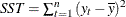, where
is the series mean.

SSE

The observation contains the sum of the squared residuals, uncorrected for the mean.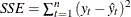, where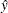is the one-step predicted value for the series.

MSE

The observation contains the mean squared error, calculated from one-step-ahead forecasts.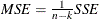, where k is the number of parameters in the model.

RMSE

The observation contains the root mean squared error.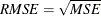.

MAPE

The observation contains the mean absolute percent error.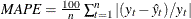.

MPE

The observation contains the mean percent error.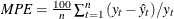.

MAE

The observation contains the mean absolute error.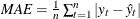.

ME

The observation contains the mean error.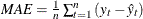.

MAXE

The observation contains the maximum error (the largest residual).

MINE

The observation contains the minimum error (the smallest residual).

MAXPE

The observation contains the maximum percent error.

MINPE

The observation contains the minimum percent error.

RSQUARE

The observation contains the R square statistic,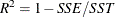. If the model fits the series badly, the model error sum of squares SSE might be larger than SST and the R square statistic will be negative.

The observation contains the adjusted R square statistic.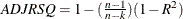.

ARSQ

The observation contains Amemiya’s adjusted R square statistic.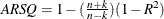.

RW_RSQ

The observation contains the random walk R square statistic (Harvey’s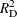statistic that uses the random walk model for comparison).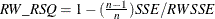,

where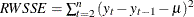and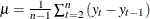.

AIC

The observation contains Akaike’s information criterion.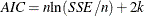.

SBC

The observation contains Schwarz’s Bayesian criterion.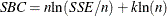.

APC

The observation contains Amemiya’s prediction criterion.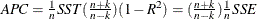.

CORR

The observation contains the correlation coefficient between the actual values and the one-step-ahead predicted values.

THEILU

The observation contains Theil’s U statistic that uses original units. See Maddala (1977, pp. 344–345), and Pindyck and Rubinfeld (1981, pp. 364–365) for more information about Theil statistics.

RTHEILU

The observation contains Theil’s U statistic calculated using relative changes.

THEILUM

The observation contains the bias proportion of Theil’s U statistic.

THEILUS

The observation contains the variance proportion of Theil’s U statistic.

THEILUC

The observation contains the covariance proportion of Theil’s U statistic.

THEILUR

The observation contains the regression proportion of Theil’s U statistic.

THEILUD

The observation contains the disturbance proportion of Theil’s U statistic.

RTHEILUM

The observation contains the bias proportion of Theil’s U statistic, calculated by using relative changes.

RTHEILUS

The observation contains the variance proportion of Theil’s U statistic, calculated by using relative changes.

RTHEILUC

The observation contains the covariance proportion of Theil’s U statistic, calculated by using relative changes.

RTHEILUR

The observation contains the regression proportion of Theil’s U statistic, calculated by using relative changes.

RTHEILUD

The observation contains the disturbance proportion of Theil’s U statistic, calculated by using relative changes.